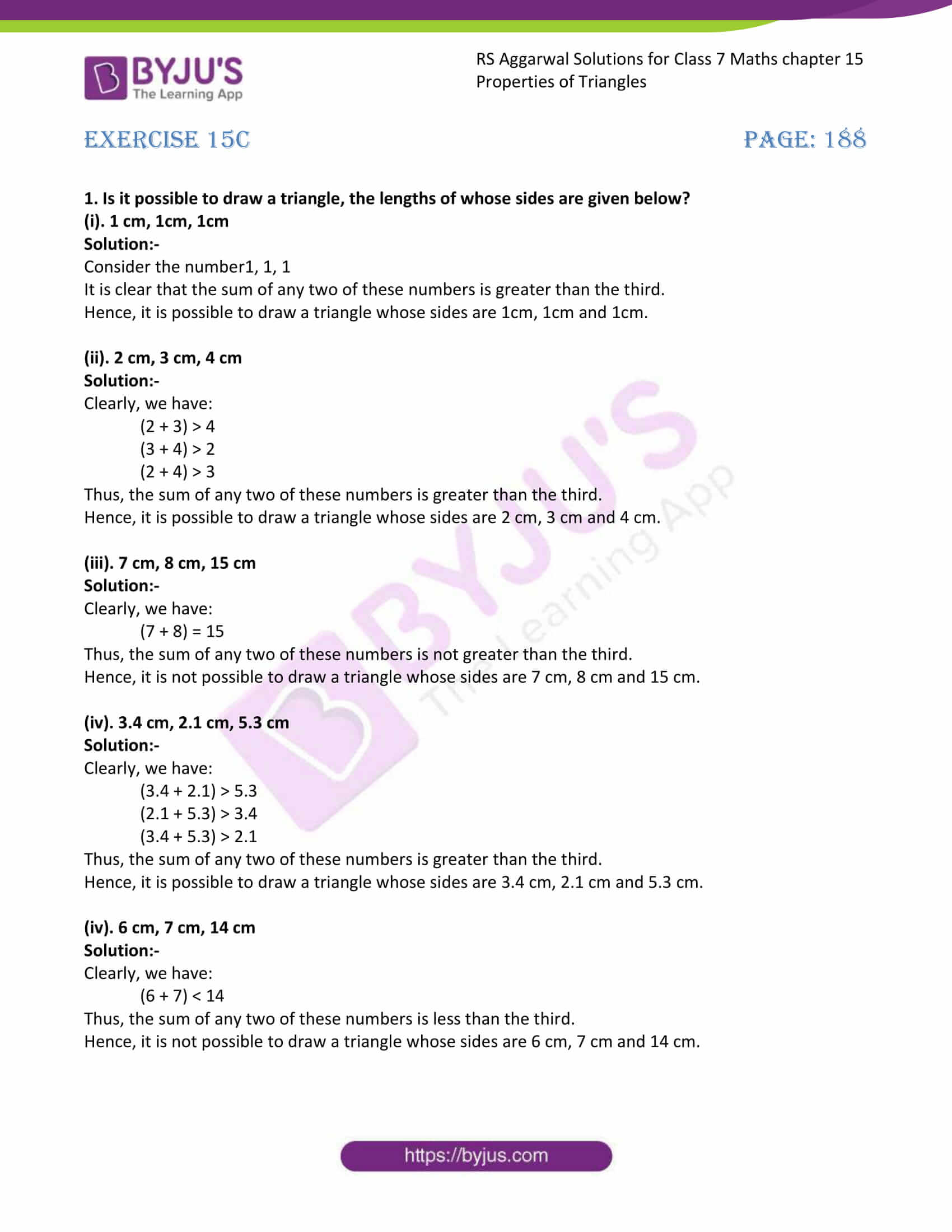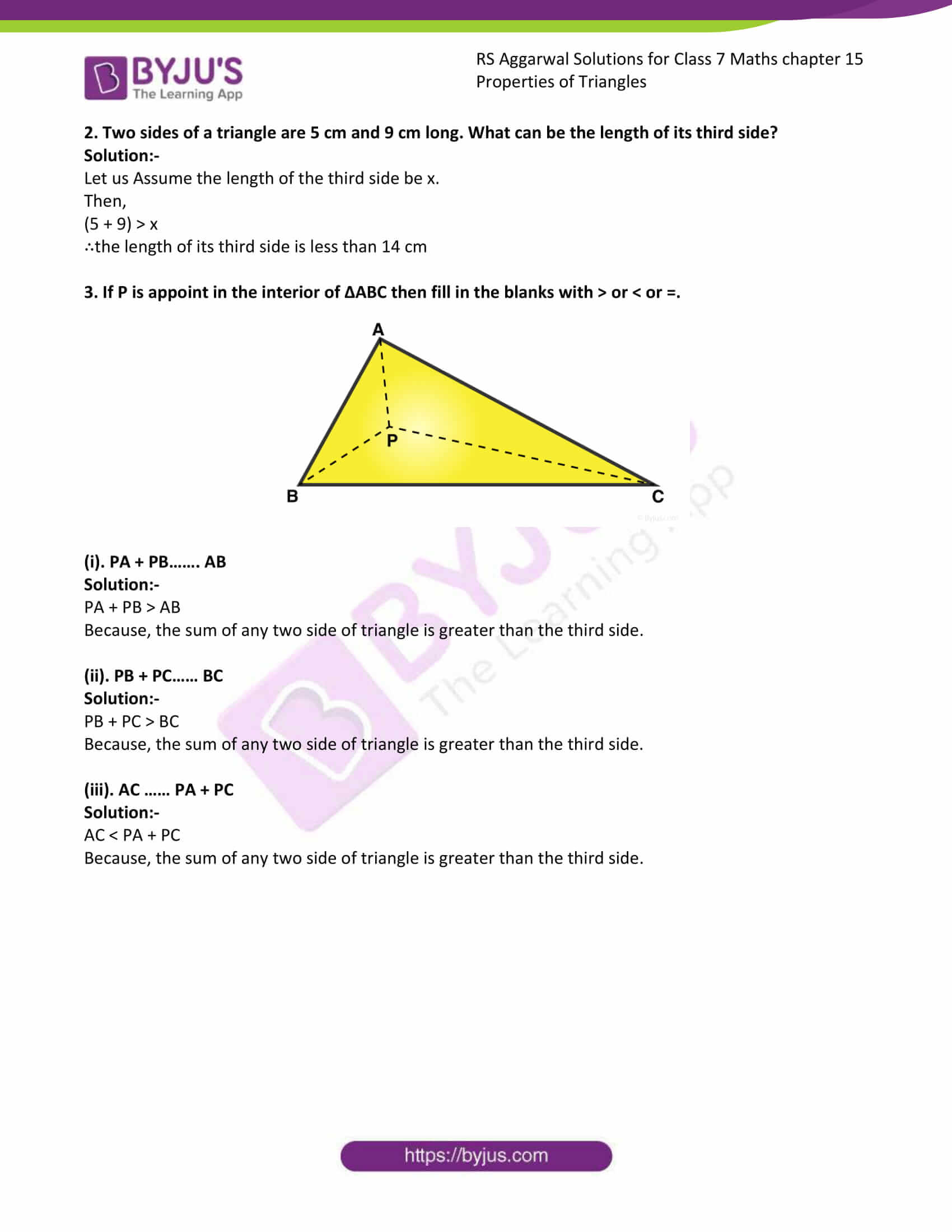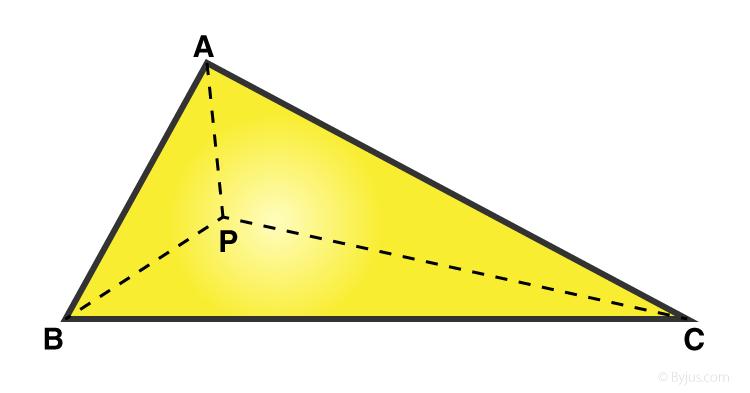# RS Aggarwal Solutions for Class 7 Maths Exercise 15C Chapter 15 Properties of Triangles

RS Aggarwal Solutions for Class 7 Maths Exercise 15C Chapter 15 Properties of Triangles, Download PDF are given here. This exercise of RS Aggarwal Solutions for Class 7 Maths Chapter 15 Properties of Triangles, is prepared in such a way that students who are in 7th standard can grasp the concepts quickly and prepare themselves very well for exams to score high marks. This exercise has the only one topic that is triangle inequality. Students can score more marks in Maths by practising RS Aggarwal Solutions for Class 7 Maths.

## Download the PDF of RS Aggarwal Solutions For Class 7 Maths Chapter 15 Properties of Triangles – Exercise 15C### Access answers to Maths RS Aggarwal Solutions for Class 7 Chapter 15 – Properties of Triangles Exercise 15C

1. Is it possible to draw a triangle, the lengths of whose sides are given below?

(i). 1 cm, 1cm, 1cm

Solution:-

Consider the number1, 1, 1

It is clear that the sum of any two of these numbers is greater than the third.

Hence, it is possible to draw a triangle whose sides are 1cm, 1cm and 1cm.

(ii). 2 cm, 3 cm, 4 cm

Solution:-

Clearly, we have:

(2 + 3) > 4

(3 + 4) > 2

(2 + 4) > 3

Thus, the sum of any two of these numbers is greater than the third.

Hence, it is possible to draw a triangle whose sides are 2 cm, 3 cm and 4 cm.

(iii). 7 cm, 8 cm, 15 cm

Solution:-

Clearly, we have:

(7 + 8) = 15

Thus, the sum of any two of these numbers is not greater than the third.

Hence, it is not possible to draw a triangle whose sides are 7 cm, 8 cm and 15 cm.

(iv). 3.4 cm, 2.1 cm, 5.3 cm

Solution:-

Clearly, we have:

(3.4 + 2.1) > 5.3

(2.1 + 5.3) > 3.4

(3.4 + 5.3) > 2.1

Thus, the sum of any two of these numbers is greater than the third.

Hence, it is possible to draw a triangle whose sides are 3.4 cm, 2.1 cm and 5.3 cm.

(iv). 6 cm, 7 cm, 14 cm

Solution:-

Clearly, we have:

(6 + 7) < 14

Thus, the sum of any two of these numbers is less than the third.

Hence, it is not possible to draw a triangle whose sides are 6 cm, 7 cm and 14 cm.

2. Two sides of a triangle are 5 cm and 9 cm long. What can be the length of its third side?

Solution:-

Let us Assume the length of the third side be x.

Then,

(5 + 9) > x

∴the length of its third side is less than 14 cm

3. If P is appoint in the interior of ΔABC then fill in the blanks with > or < or =.(i). PA + PB……. AB

Solution:-

PA + PB > AB

Because, the sum of any two side of triangle is greater than the third side.

(ii). PB + PC…… BC

Solution:-

PB + PC > BC

Because, the sum of any two side of triangle is greater than the third side.

(iii). AC …… PA + PC

Solution:-

AC < PA + PC

Because, the sum of any two side of triangle is greater than the third side.

### Access other exercises of RS Aggarwal Solutions For Class 7 Chapter 15 – Properties of Triangles

Exercise 15A Solutions

Exercise 15B Solutions

Exercise 15D Solution